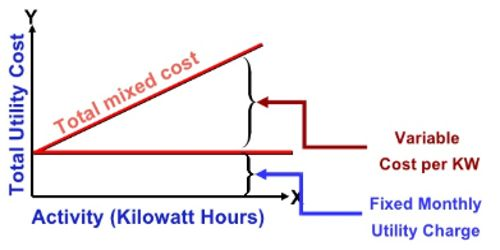Accounting

# Distinguish between Variable, Fixed, and Mixed Cost.Distinguish between Variable, Fixed, and Mixed Cost

Variable Cost: Changes in total, in direct proportion to changes in the level of activity. The total cost increases/decreases as units made increases/decreases. Variable cost is constant if expressed on a per unit basis. Direct material, direct labor, and variable overhead are all variable costs. Costs that vary with sales, such as sales commission are variable costs. It is a variable cost if it costs you more if you make or sell one more. A common example of a variable cost is the direct materials cost.

Fixed Cost: Total cost does not change with changes in the volume of activity (within a relevant range). The cost per unit will change as the number of units change. Rent, insurance, administrative salaries are examples of fixed costs. These costs do not change just because you make or sell one more unit as long as you stay within the relevant range. A common example of a fixed cost is rent.Mixed Costs (sometimes called semi-variable – A cost that has the characteristics of both variable and fixed cost is called mixed or semi-variable cost.

• One that contains both variable and fixed costs elements;
• Fixed – a minimum cost of having a service ready and available for use,
• Variable – cost incurred for actual consumption of the service,
• Total Mixed Costs = Total Fixed Cost \$ + (Variable Cost \$ per activity x # of the activity).